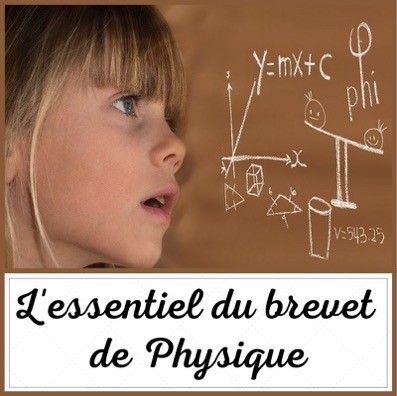# PHYSICAL PATENT: THE ESSENTIAL

We have gathered below the main formulas of the Physics patent.

I. MASS-WEIGHT:

I.1. The density :

La Volumic mass, also called density density of mass, is a physical quantity which characterizes the mass of this substance per unit volume.where r (density) is in kg / m3 or kg.m-3; m (mass) is in kg or g; V (volume) is in m3 or cm3

By extension :

Examples : 1 cone has a mass (m) of 250 g and a volume (V) of 20 cm3. Its density is m / V = ​​250/20 = 12,5 g.cm3

I.2. The weight value:

Le weight of a body (mass m) Is the force of gravity exerted on it.

where P (the weight) is in N (Newton); M (mass) is in kg or g; G (the intensity or force of gravity) is in N / kg or N. kg-1

By extension :

Examples : A man has a mass of 70 kg. The intensity of gravity on Earth is 10 N.kg-1 . The value of the weight of the man is 70 times 10 or 700 N

Quote on physics:

“Physics gives the how much, metaphysics the how.”

Buffon

Continuation of the article, speed and kinetic energy, page 2